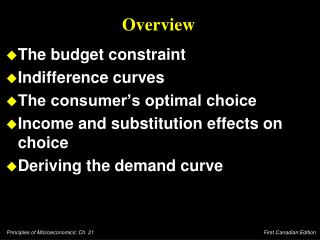# Overview - PowerPoint PPT PresentationDownload PresentationOverview

Overview
Download Presentation## Overview

- - - - - - - - - - - - - - - - - - - - - - - - - - - E N D - - - - - - - - - - - - - - - - - - - - - - - - - - -
##### Presentation Transcript

1. Overview • The budget constraint • Indifference curves • The consumer’s optimal choice • Income and substitution effects on choice • Deriving the demand curve

2. The Budget Constraint:What Consumers Can Afford “The budget constraint depicts the consumption possibilities available to the individual.” • People consume less than they desire because their spending is constrained, or limited, by their income.

3. The Budget Constraint Pepsi Pepsi (\$2) 500 Pizza Pizza (\$10) 100

4. The Budget Constraint Pepsi (\$2) Any point on the constraint line equals \$1,000, the income available to spend on the two products. B 500 C 250 A Pizza (\$10) 50 100

5. The Budget Constraint • The slope of the budget constraint measures the rate at which the consumer can trade one good for the other. • The slope equals the relative price of the two goods, i.e. the price of one good compared to the price of the other.

6. Indifference Curves Pepsi . C . . B I2 I1 A Pizza

7. Indifference Curves • The consumer is indifferent among combinations A, B, and C, because they are all on the same curve. • The slope at any point on an indifference curve equals the rate at which the consumer is willing to substitute one good for the other.

8. Indifference Curves Pepsi Slope between points A and B. Tradeoff between the two bundles. . C . . . B D I2 I1 A Pizza

9. The Marginal Rate of Substitution • The slope is called the marginal rate of substitution. • The rate at which consumers are willing to trade one good for another. • The amount that the consumer must receive as compensation in order to give up something else that he/she desires.

10. Properties of Indifference Curves • Higher indifference curves are preferred to lower ones. • Indifference curves are downward sloping. • Indifference curves do not cross. • Indifference curves are bowed inward.

11. Optimization: What the Consumer Chooses • Consumers would like to obtain the combination of goods on the highest possible indifference curve. However, the budget constraint may restrict or limit the consumer to a lower indifference curve. • Combining the indifference curve and budget constraint determines the optimum choice.

12. The Consumer’s Optimal Choice Pepsi Consumer’s indifference curves, based on personal preferences. I3 I2 I1 Pizza

13. The Consumer’s Optimal Choice Pepsi Consumer’s budget constraint. I3 I2 I1 Pizza

14. The Consumer’s Optimal Choice • The point at which the indifference curve and the budget constraint touch (i.e. its tangent) is called the optimum. • The consumer chooses consumption of the two goods so that the marginal rate of substitution equals the relative price.

15. A Change in Income Affects Choices Pepsi An Increase in income shifts the budget constraint. . QPepsi I3 I2 I1 Pizza QPizza

16. A Change in Income Affects Choices Pepsi A new optimum . QNew QPepsi I3 I2 I1 Pizza QPizza QNew

17. Changes in Prices Affect Consumer’s Choices • A fall in the price of any good will shift the budget constraint outward and will change the slope of the budget constraint.

18. Deriving the Demand Curve A consumer’s demand curve is a summary of the optimal decisions that arise from his budget constraint and indifference curves.

19. Conclusion • Indifference curve analysis describes how individuals make decisions. It has many relevant applications. • If people behave AS IF they followed the model, then the model will yield accurate and useful predictions and results.